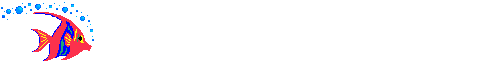# Elementary Algebra Assignment #391. The length of a rectangle is 2 times the width. The area is 32 square inches. Find the dimensions of the rectangle. 2. A market sells regular coffee for \$3 a pound and a gourmet coffee for \$5 a pound. The two types are mixed to create 100 pounds of coffee selling for \$4.50 a pound. How many pounds of each type of coffee should be used in the mixture? 3. Tickets for a concert sold for \$11 and \$8. If 600 tickets were sold for one evening and the receipts were \$5550, how many of each kind of ticket were sold?4. A chemist is mixing a 15% acid solution with a 60% acid solution. How many milliliters of the stronger solution must be mixed with the weaker to produce 200 milliliters of a 33% solution? 5. Christine ran 4 times as many miles as Lora, while Valerie ran 2 miles less than Lora. If the three ran a total of 16 miles, how far did Christine go? 6. The length of a rectangle is 2 cm less than 3 times its width. If the area of the rectangle is 40cm2, find the dimensions of the rectangle.